# 【机器学习】确定最佳聚类数目的10种方法

library(gclus)
data(wine)

Loading required package: cluster因为我们要找一个数据集进行聚类分析，所以不需要第一列的种类标签信息，因此去掉第一列。

dataset <- wine[,-1] #去除分类标签
dataset <- scale(dataset)


## 判定方法

### 1.mclust包

mclust包是聚类分析非常强大的一个包，也是上课时老师给我们介绍的一个包，每次导入时有一种科技感 :) 帮助文档非常详尽，可以进行聚类、分类、密度分析
Mclust包方法有点“暴力”，聚类数目自定义，比如我选取的从1到20，然后一共14种模型，每一种模型都计算聚类数目从1到20的BIC值，最终确定最佳聚类数目，这种方法的思想很直接了当，但是弊端也就显然易见了——时间复杂度太高，效率低。

library(mclust)
m_clust <- Mclust(as.matrix(dataset), G=1:20) #聚类数目从1一直试到20
summary(m_clust)

Gaussian finite mixture model fitted by EM algorithm

Mclust EVE (ellipsoidal, equal volume and orientation) model with 3 components:

log.likelihood   n  df       BIC       ICL
-3032.45 178 156 -6873.257 -6873.549

Clustering table:
1  2  3


63 51 64

plot(m_clust, "BIC")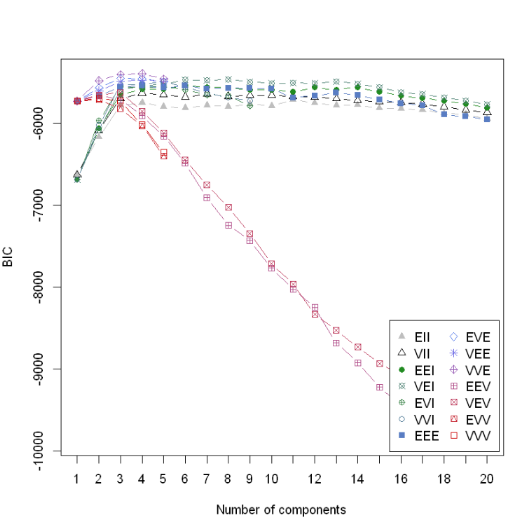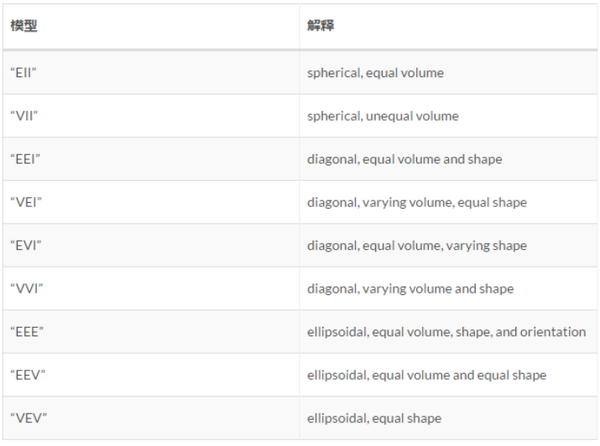mclust包还可以用于分类、密度估计等，这个包值得好好把玩。

1.维基上的贝叶斯信息准则定义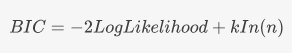2.Mclust包中的BIC定义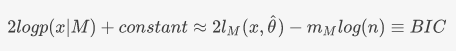这是Mclust包里面作者定义的“BIC值”，此BIC非彼BIC，这里是作者自己定义的BIC，可以看到，这里的BIC与极大似然估计是成正比的，所以这里是BIC值越大越好，与贝叶斯信息准则值越小模型越好的结论并不冲突

### 2.Nbclust包

Nbclust包是我在《R语言实战》上看到的一个包，思想和mclust包比较相近，也是定义了几十个评估指标，然后聚类数目从2遍历到15（自己设定），然后通过这些指标看分别在聚类数为多少时达到最优，最后选择指标支持数最多的聚类数目就是最佳聚类数目。

library(NbClust)
set.seed(1234) #因为method选择的是kmeans，所以如果不设定种子，每次跑得结果可能不同
nb_clust <- NbClust(dataset,  distance = "euclidean",
min.nc=2, max.nc=15, method = "kmeans",
index = "alllong", alphaBeale = 0.1)

*** : The Hubert index is a graphical method of determining the number of clusters.
In the plot of Hubert index, we seek a significant knee that corresponds to a
significant increase of the value of the measure i.e the significant peak in Hubert
index second differences plot.

*** : The D index is a graphical method of determining the number of clusters.
In the plot of D index, we seek a significant knee (the significant peak in Dindex
second differences plot) that corresponds to a significant increase of the value of
the measure.

*******************************************************************
* Among all indices:
* 5 proposed 2 as the best number of clusters
* 16 proposed 3 as the best number of clusters
* 1 proposed 10 as the best number of clusters
* 1 proposed 12 as the best number of clusters
* 1 proposed 14 as the best number of clusters
* 3 proposed 15 as the best number of clusters

***** Conclusion *****

* According to the majority rule, the best number of clusters is  3

*******************************************************************

barplot(table(nb_clust$Best.nc[1,]),xlab = "聚类数",ylab = "支持指标数")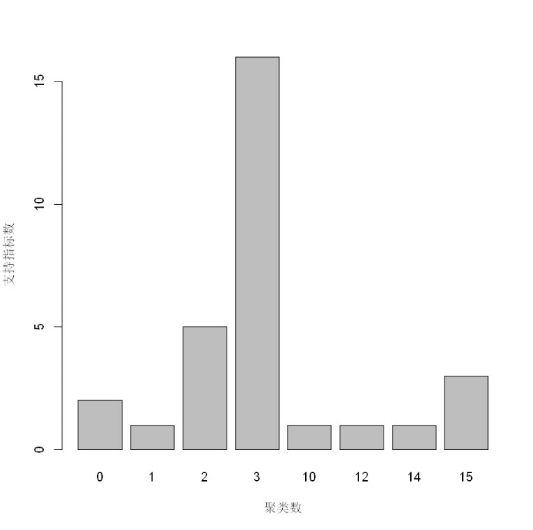可以看到有16个指标支持最佳聚类数目为3，5个指标支持聚类数为2，所以该方法推荐的最佳聚类数目为3. ### 3. 组内平方误差和——拐点图 想必之前动辄几十个指标，这里就用一个最简单的指标——sum of squared error (SSE)组内平方误差和来确定最佳聚类数目。这个方法也是出于《R语言实战》，自定义的一个求组内误差平方和的函数。 wssplot <- function(data, nc=15, seed=1234){ wss <- (nrow(data)-1)*sum(apply(data,2,var)) for (i in 2:nc){ set.seed(seed) wss[i] <- sum(kmeans(data, centers=i)$withinss)
}
plot(1:nc, wss, type="b", xlab="Number of Clusters",
ylab="Within groups sum of squares")}

wssplot(dataset)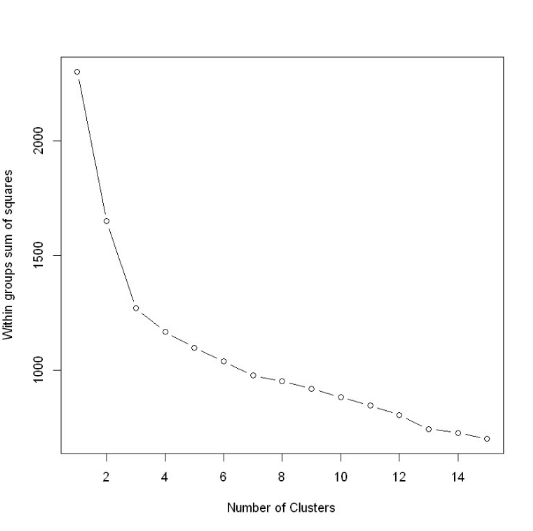library(factoextra)
library(ggplot2)
set.seed(1234)
fviz_nbclust(dataset, kmeans, method = "wss") +
geom_vline(xintercept = 3, linetype = 2)

Loading required package: ggplot2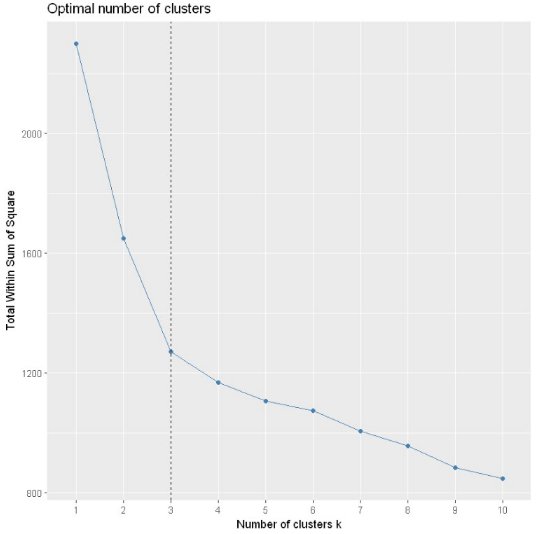km.res <- kmeans(dataset,3)
fviz_cluster(km.res, data = dataset)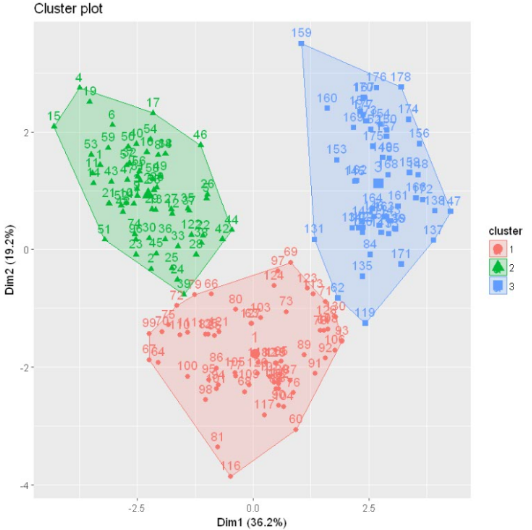### 4. PAM(Partitioning Around Medoids) 围绕中心点的分割算法

k-means算法取得是均值，那么对于异常点其实对其的影响非常大，很可能这种孤立的点就聚为一类，一个改进的方法就是PAM算法，也叫k-medoids clustering

library(fpc)
pamk.best <- pamk(dataset)
pamk.best$nc  3 pamk函数不需要提供聚类数目，也会直接自动计算出最佳聚类数，这里也得到为3 得到聚类数提供给cluster包下的pam函数并进行可视化 library(cluster) clusplot(pam(dataset, pamk.best$nc))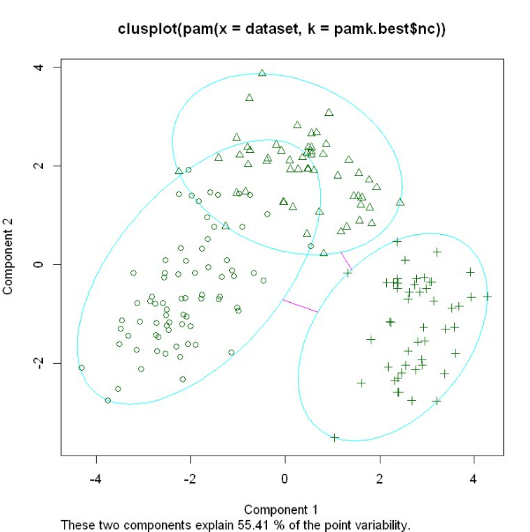### 5.Calinsky criterion

library(vegan)
ca_clust <- cascadeKM(dataset, 1, 10, iter = 1000)
ca_clust$results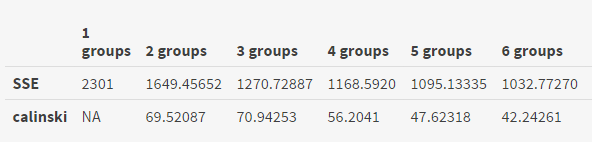可以看到该函数把组内平方和误差和Calinsky都计算出来了，可以看到calinski在聚类数为3时达到最大值。 calinski.best <- as.numeric(which.max(ca_clust$results[2,]))
calinski.best


3

plot(fit, sortg = TRUE, grpmts.plot = TRUE)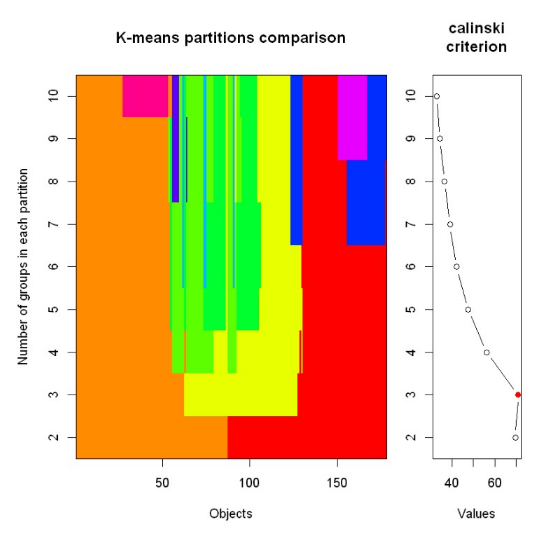calinski<-as.data.frame(ca_clust$results[2,]) calinski$cluster <- c(1:10)
library(ggplot2)
ggplot(calinski,aes(x = calinski[,2], y = calinski[,1]))+geom_line()

Warning message:
"Removed 1 rows containing missing values (geom_path)."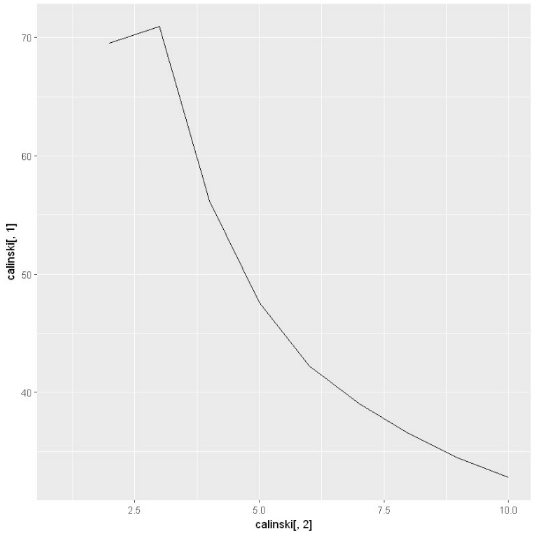### 6.Affinity propagation (AP) clustering

AP算法的基本思想是将全部样本看作网络的节点，然后通过网络中各条边的消息传递计算出各样本的聚类中心。聚类过程中，共有两种消息在各节点间传递，分别是吸引度( responsibility)和归属度(availability) 。AP算法通过迭代过程不断更新每一个点的吸引度和归属度值，直到产生m个高质量的Exemplar（类似于质心），同时将其余的数据点分配到相应的聚类中

library(apcluster)
ap_clust <- apcluster(negDistMat(r=2), dataset)
length(ap_clust@clusters)


15

heatmap(ap_clust)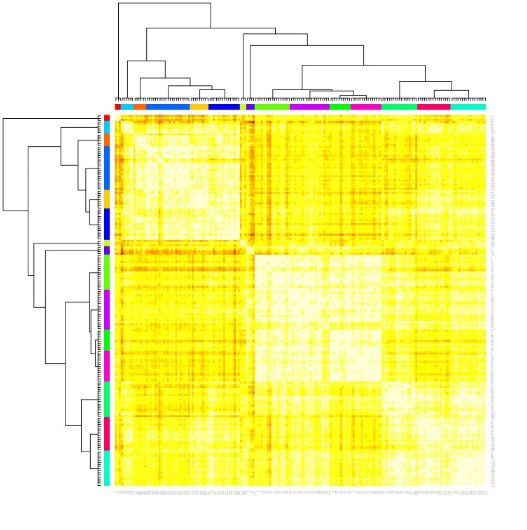### 7. 轮廓系数Average silhouette method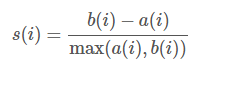a(i)是测量组内的相似度,b(i)是测量组间的相似度，s(i)范围从-1到1，值越大说明组内吻合越高，组间距离越远——也就是说，轮廓系数值越大，聚类效果越好

require(cluster)
library(factoextra)
fviz_nbclust(dataset, kmeans, method = "silhouette")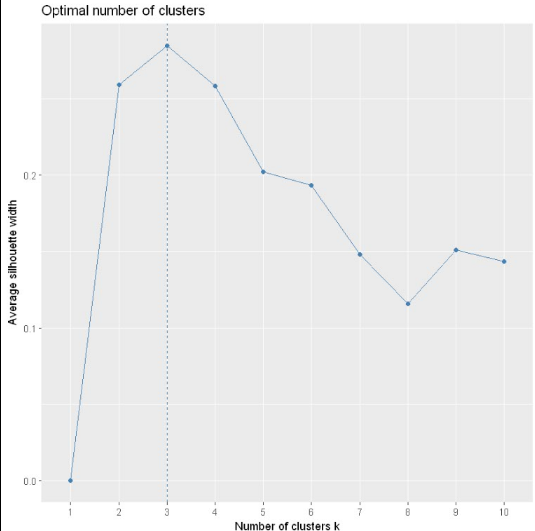### 8. Gap Statistic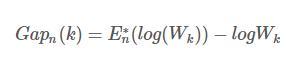library(cluster)
set.seed(123)
gap_clust <- clusGap(dataset, kmeans, 10, B = 500, verbose = interactive())
gap_clust

Clustering Gap statistic ["clusGap"] from call:
clusGap(x = dataset, FUNcluster = kmeans, K.max = 10, B = 500,     verbose = interactive())
B=500 simulated reference sets, k = 1..10; spaceH0="scaledPCA"
--> Number of clusters (method 'firstSEmax', SE.factor=1): 3
logW   E.logW       gap     SE.sim
[1,] 5.377557 5.863690 0.4861333 0.01273873
[2,] 5.203502 5.758276 0.5547745 0.01420766
[3,] 5.066921 5.697322 0.6304006 0.01278909
[4,] 5.023936 5.651618 0.6276814 0.01243239
[5,] 4.993720 5.615174 0.6214536 0.01251765
[6,] 4.962933 5.584564 0.6216311 0.01165595
[7,] 4.943241 5.556310 0.6130690 0.01181831
[8,] 4.915582 5.531834 0.6162518 0.01139207
[9,] 4.881449 5.508514 0.6270646 0.01169532
[10,] 4.855837 5.487005 0.6311683 0.01198264

library(factoextra)
fviz_gap_stat(gap_clust)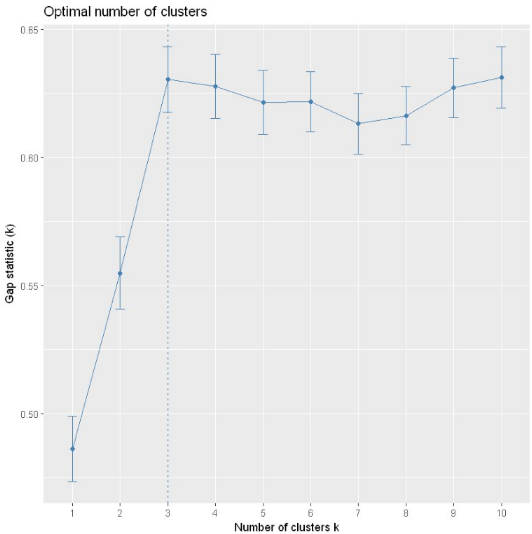### 9.层次聚类

h_dist <- dist(as.matrix(dataset))
h_clust<-hclust(h_dist)
plot(h_clust, hang = -1, labels = FALSE)
rect.hclust(h_clust,3)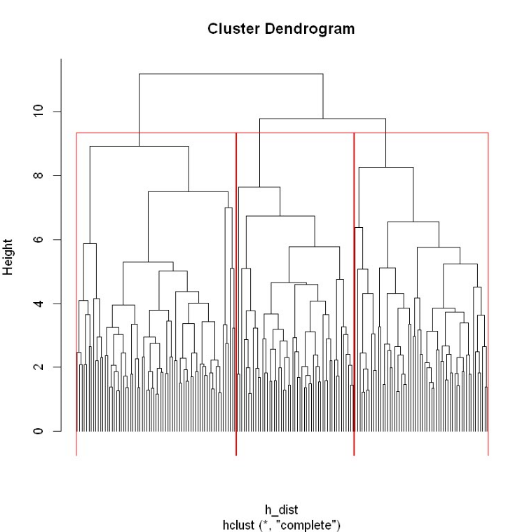### 10.clustergram

clustergram(dataset, k.range = 2:8, line.width = 0.004)

Loading required package: colorspace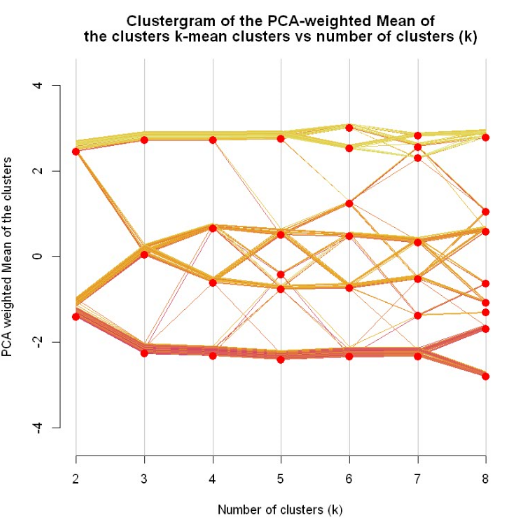## 方法汇总与比较

wine数据集我们知道其实是分为3类的，以上10种判定方法中：

1. 层次聚类和clustergram方法、肘点图法，需要人工判定，虽然可以得出大致的最佳聚类数，但算法本身不会给出最佳聚类数
2. 除了Affinity propagation (AP) clustering 给出最佳聚类数为15，剩下6种全都是给出最佳聚类数为3
3. 选用上次文本挖掘的矩阵进行分析(667*1623)
• mclust效果很差，14种模型只有6种有结果
• bclust报错
• SSE可以运行
• fpc包中的pamk函数聚成2类，明显不行
• Calinsky criterion聚成2类
• Affinity propagation (AP) clustering 聚成28类，相对靠谱
• 轮廓系数Average silhouette聚类2类
• gap-Statistic跑不出结果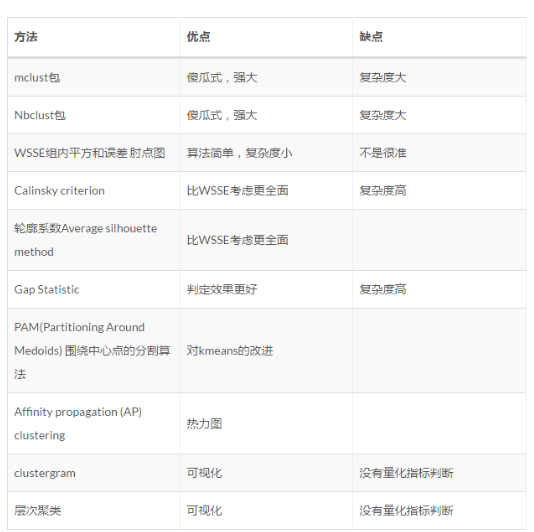R语言实战第二版
Partitioning cluster analysis: Quick start guide - Unsupervised Machine Learning
BIC
Cluster analysis in R: determine the optimal number of clusters
Calinski-Harabasz CriterionCalinski-Harabasz criterion clustering evaluation object
Determining the optimal number of clusters: 3 must known methods - Unsupervised Machine Learning
 affinity-propagation聚类算法Affinity Propagation(AP)
轮廓系数https://en.wikipedia.org/wiki/Silhouette(clustering))
gap statistic-Tibshirani R, Walther G, Hastie T. Estimating the number of clusters in a data set via the gap statistic[J]. Journal of the Royal Statistical Society: Series B (Statistical Methodology), 2001, 63(2): 411-423.

posted on 2017-07-07 19:08  曹明  阅读(32974)  评论(3编辑  收藏